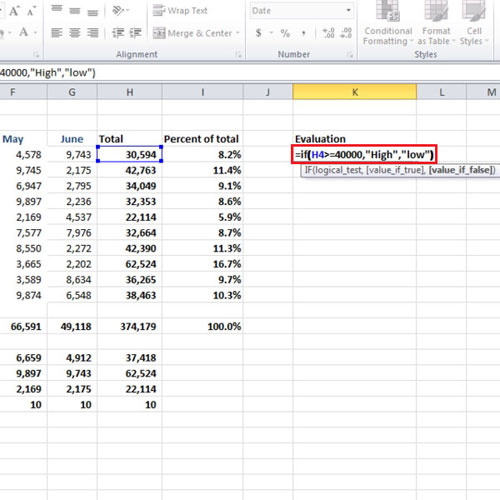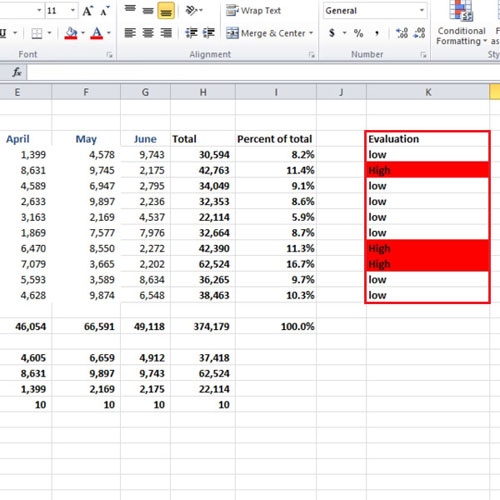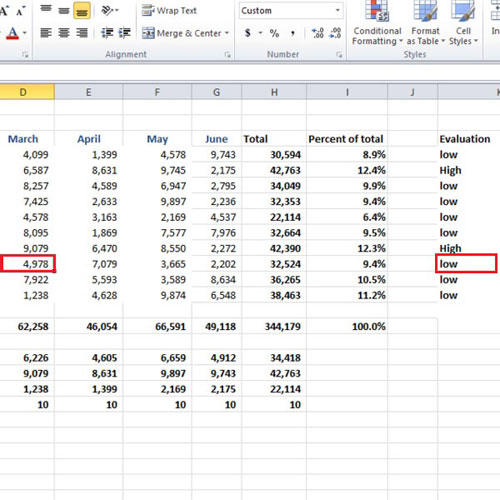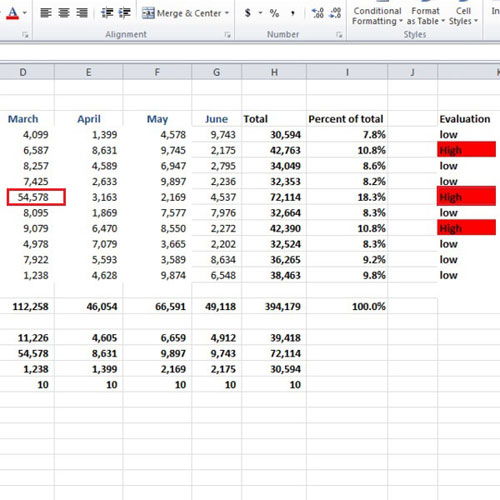## How to Use IF Function in Excel 2010

In Excel, there are more than 300 functions. There is one called the “if” function. It allows you to apply logical reasoning into the data and by that you can perform various tasks. It allows you to test an argument; the argument can either be true or false. We can apply a condition which will be tested. If it is true or false, different results will be shown accordingly. Such kind of logical tests can be applied by using the “if” function.

Follow this step by step tutorial to learn how to use if function in excel.

Step # 1 – Use the IF function

In this worksheet we have values for the expenses that were incurred; we want to write “high” if the total value exceeds \$40,000, otherwise we want to display “low”. Thus we need to insert a condition. In a new column, write “Evaluation” and under that write “=if(h4>=40000,”high”,”low”)”. This logical test argues that if the value in cell “h4”, which is the total of all the expenses incurred during the 6 months period in New York City, is greater than “\$40000”, then write “High”, otherwise write “Low”.Step # 2 – Evaluate the rest of the cities

After writing the function in Excel, press the “enter” key and use the auto fill handle to apply the function for the rest of the cities. In this tutorial, only three cities are showing “high” value and the rest of them are showing “low” value in the “Evaluation” column. The cities “Los Angeles”, “Washington D.C” and “Miami” have totals which exceed the limit which is \$40,000.Step # 3 – Change the values

In this tutorial, we changed the values in cell “d11”, and “d8”. In cell “d11”, we changed it to around 5 thousand so that the total may drop and “low” will be shown in front of it.
In cell “d8”, we increased the value so that “high” will be written.Step # 4 – Clicking on All items

After you make the changes, you will see that “high” and “low” will also change depending on the total value. As soon as you will change the values in the cells, the logical test in the “if” function will be applied to the new total and true & false reasoning will be applied to the totals.